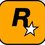# Help please

$\huge\int \dfrac{\cos^5 x+\cos^4 x}{1-2\cos 3x}$Note by Rohit Ner
5 years, 9 months ago

This discussion board is a place to discuss our Daily Challenges and the math and science related to those challenges. Explanations are more than just a solution — they should explain the steps and thinking strategies that you used to obtain the solution. Comments should further the discussion of math and science.

When posting on Brilliant:

• Use the emojis to react to an explanation, whether you're congratulating a job well done , or just really confused .
• Ask specific questions about the challenge or the steps in somebody's explanation. Well-posed questions can add a lot to the discussion, but posting "I don't understand!" doesn't help anyone.
• Try to contribute something new to the discussion, whether it is an extension, generalization or other idea related to the challenge.
• Stay on topic — we're all here to learn more about math and science, not to hear about your favorite get-rich-quick scheme or current world events.

MarkdownAppears as
*italics* or _italics_ italics
**bold** or __bold__ bold
- bulleted- list
• bulleted
• list
1. numbered2. list
1. numbered
2. list
Note: you must add a full line of space before and after lists for them to show up correctly
paragraph 1paragraph 2

paragraph 1

paragraph 2

[example link](https://brilliant.org)example link
> This is a quote
This is a quote
    # I indented these lines
# 4 spaces, and now they show
# up as a code block.

print "hello world"
# I indented these lines
# 4 spaces, and now they show
# up as a code block.

print "hello world"
MathAppears as
Remember to wrap math in $$ ... $$ or $ ... $ to ensure proper formatting.
2 \times 3 $2 \times 3$
2^{34} $2^{34}$
a_{i-1} $a_{i-1}$
\frac{2}{3} $\frac{2}{3}$
\sqrt{2} $\sqrt{2}$
\sum_{i=1}^3 $\sum_{i=1}^3$
\sin \theta $\sin \theta$
\boxed{123} $\boxed{123}$

## Comments

Sort by:

Top Newest

I am getting a nice closed form if the numerator is $\cos 5x + \cos 4x$. I think if it is a JEE problem then the numerator should be what I have written. Can you please check? Otherwise I'll try with this again.

- 5 years, 9 months ago

Log in to reply

I am sure about the question. The reason I put the jee tag was to get to know if there are any methods of jee applicable.

- 5 years, 9 months ago

Log in to reply

What makes you think that it has a closed form?

- 5 years, 9 months ago

Log in to reply

This problem was given to me by my class mate. I tried every integration technique possible. Even I doubt if there exists a valid closed form for it. Please help me sir.

- 5 years, 9 months ago

Log in to reply

There's no simple closed form without using hypergeometric functions. Apply $\cos(3x) /\cos(2x) = 1 - 2\cos(2x)$ and reducing the powers of trigonometric functions to 1 shows that we are essentially solving for at least one of $\int \sin(ax) \csc(bx) dx$ , $\int \sin(ax) \sec(bx) dx$, $\int \cos(ax) \sec(bx) dx$, $\int \cos(ax) \csc(bx) dx$ which can't be stated in terms of elementary functions because for all of these cases, $b \ne 1$.

- 5 years, 9 months ago

Log in to reply

I know a method but haven't done it yet.

1. Write $\cos^5(x)$ and $\cos^4(x)$ as $\frac{1}{16}(10 \cos(x) + 5 \cos(3x) + \cos(5x))$ and $\frac{1}{8}(3 + 4 \cos(2x) + \cos(4x))$.

2. Substitute $z = {e}^{ix}$.

3. Then, you would get a rational polynomial function in terms of z which can "easily" be solved using Partial Fraction or Division approach.

I know this method is way too tedious but that's the most general way to tackle these types of problems.

- 5 years, 9 months ago

Log in to reply

You can't solve it by simple Partial Fractions because the denominator of this function does not have any "nice" roots.

- 5 years, 9 months ago

Log in to reply

Oh! I see. I hadn't checked it so I might be wrong. If it doesn't have "nice" roots then also it doesn't matter, computer will do it, it doesn't discriminate b/w real and complex :P

- 5 years, 9 months ago

Log in to reply

We might have different opinion of "nice" closed form. Does the integration of $\frac1{x^3 + 3x^2 + 5x+7}$ have a nice form?

- 5 years, 9 months ago

Log in to reply

That's quite nice! :P For me, everything has a nice "closed"(I didn't use this word originally) form. Even the error function does! :P

- 5 years, 9 months ago

Log in to reply

To me, even the error function is not "nice". I only consider elementary functions to be "nice".

If you consider all of these to have a "nice" closed form, then it's hard to judge whether an integral is worth solving or not. Don't you think so?

- 5 years, 9 months ago

Log in to reply

I don't think this is an IITJEE question. This question is not integrable to our knowledge(atleast till JEE point of view). You can use higher level integration to solve this.

- 5 years, 7 months ago

Log in to reply

×

Problem Loading...

Note Loading...

Set Loading...